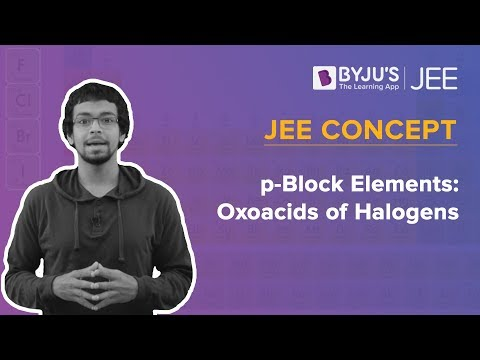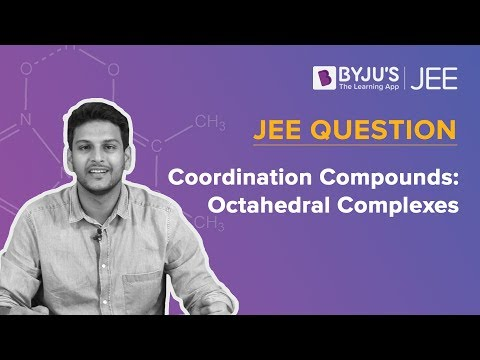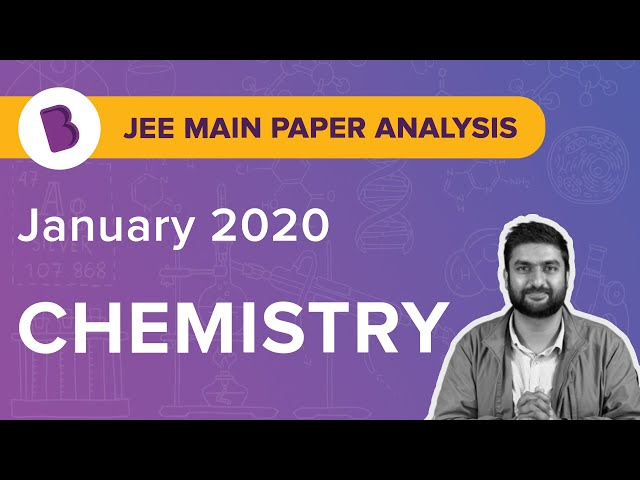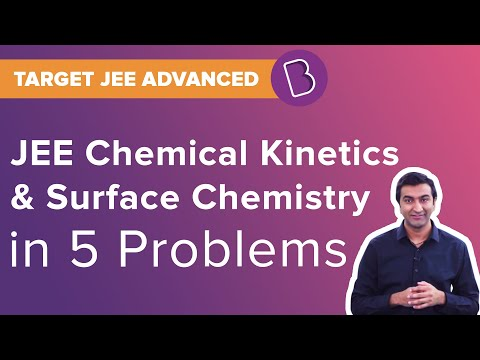Win up to 100% scholarship on Aakash BYJU'S JEE/NEET courses with ABNAT Win up to 100% scholarship on Aakash BYJU'S JEE/NEET courses with ABNAT

# JEE Main 2020 Chemistry Paper With Solutions Sept 3 (Shift 2)

JEE Main 2020 Paper With Solutions Chemistry – Shift 2 is a perfect resource to ace the upcoming JEE exams. Experts recommend candidates to solve JEE Main question papers before appearing for the examination. It will help the students to understand the type of questions asked, difficulty level, paper pattern and marking scheme. Solving JEE Main Previous Year Papers is very important for JEE Main preparation. It is designed to help the candidates to achieve their best scores and also assists in determining their weaker areas and identifying the gaps in exam-taking skills.
September 3 Shift 2 – Chemistry
Question 1: The five successive ionization enthalpies of an element are 800, 2427, 3658, 25024 and 32824 kJ mol–1. The number of valence electrons in the element is:
1) 2
2) 4
3) 3
4) 5

Fourth & Fifth I.E. are very high (periodic properties) indicating the presence of three valence shell electrons.

Question 2: The incorrect statement is:
1) Manganate and permanganate ions are tetrahedral
2) In manganate and permanganate ions, the -bonding takes place by the overlap of p orbitals of oxygen and d-orbitals of manganese
3) Manganate and permanganate ions are paramagnetic
4) Manganate ion is green in colour and permanganate ion is purple in colour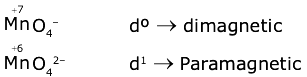Question 3: Match the following drugs with their therapeutic actions:

(i) Ranitidine (a) Antidepressant

(ii) Nardil (Phenelzine) (b) Antibiotic

(iii)Chloramphenicol (c) Antihistamine

(iv) Dimetane (Brompheniramine) (d) Antacid

(e) Analgesic

1) (i)-(d); (ii)-(a); (iii)-(b); (iv)-(c)
2) (i)-(d); (ii)-(c); (iii)-(a); (iv)-(e)
3) (i)-(a); (ii)-(c); (iii)-(b); (iv)-(e)
4) (i)-(e); (ii)-(a); (iii)-(c); (iv)-(d)

Question 4: An ionic micelle is formed on the addition of
1) liquid diethyl ether to aqueous NaCl solution
2) sodium stearate to pure toluene
3) excess water to liquid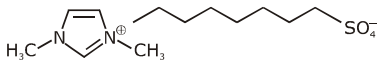4) excess water to liquid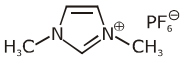The ionic micelles formed by the addition of water to soap {sodium stearate}.

Question 5: Among the statements (I–IV), the correct ones are:

(I) Be has a smaller atomic radius compared to Mg.

(II) Be has higher ionization enthalpy than Al.

(III) Charge/radius ratio of Be is greater than that of Al.

(IV) Both Be and Al form mainly covalent compounds.

1) (I), (II) and (IV)
2) (I), (II) and (III)
3) (II), (III) and (IV)
4) (I), (III) and (IV)

Refer S-Block

Question 6: Complex A has a composition of H12O6Cl3Cr. If the complex on treatment with conc. H2SO4 loses 13.5% of its original mass, the correct molecular formula of A is: [Given: the atomic mass of Cr = 52 amu and Cl = 35 amu]
1) [Cr(H2O)5Cl]Cl2 .H2O
2) [Cr(H2O)4Cl2]Cl.2H2O
3) [Cr(H2O)3Cl3].3H2O
4) [Cr(H2O)6]Cl3

Let x molecule of water are lost then

13.5 = {[x × 18] / [6 × 18 + 3 × 35 + 52]} * 100

x = 1.99 ≃ 2

Question 7: The decreasing order of reactivity of the following compounds towards nucleophilic substitution (SN2) is: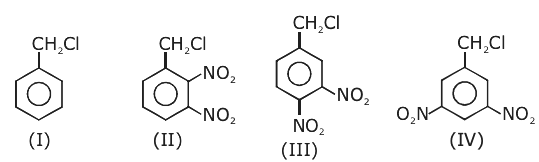1) (III) > (II) > (IV) > (I)
2) (IV) > (II) > (III) > (I)
3) (II) > (III) > (IV) > (I)
4) (II) > (III) > (I) > (IV)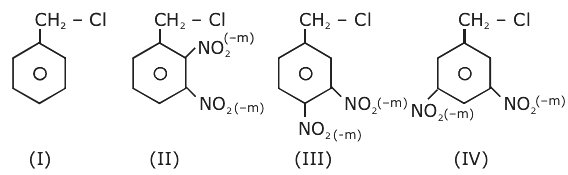Question 8: The increasing order of the reactivity of the following compounds in nucleophilic addition reaction is: Propanal, Benzaldehyde, Propanone, Butanone
1) Benzaldehyde < Propanal < Propanone < Butanone
2) Propanal < Propanone < Butanone < Benzaldehyde
3) Butanone < Propanone < Benzaldehyde < Propanal
4) Benzaldehyde < Butanone < Propanone < Propanal

Rate of Nucleophilic addition Aldehyde > Ketone Aliphatic aldehyde > Aromatic aldehyde

Question 9: The major product in the following reaction is: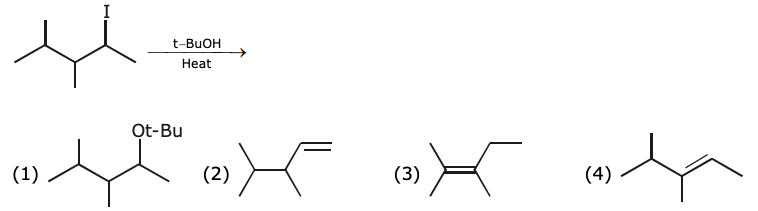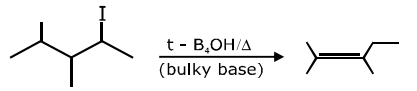Question 10: The incorrect statement(s) among (a) – (d) regarding acid rain is (are):

(a) It can corrode water pipes.

(b) It can damage structures made up of stone.

(c) It cannot cause respiratory ailments in animals

(d) It is not harmful for trees

1) (a), (b) and (d)
2) (a), (c) and (d)
3) (c) and (d)
4) (c) only

Acid rain can cause respiratory ailments in animals and also harmful to trees and plants.

Question 11: 100 mL of 0.1 M HCl is taken in a beaker and to it, 100 mL of 0.1 M NaOH is added in steps of 2 mL and the pH is continuously measured. Which of the following graphs correctly depicts the change in pH?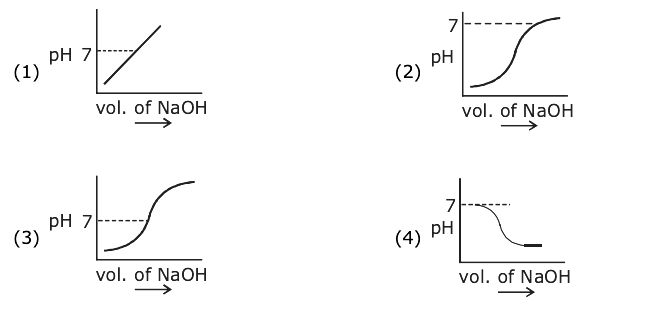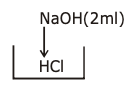initially pH will be acidic < 7

at eq pH pH = 7

& finally pH will be basic > 7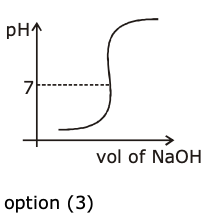Question 12: Consider the hypothetical situation where the azimuthal quantum number, l, takes values 0, 1, 2, …… n + 1, where n is the principal quantum number. Then, the element with atomic number:
1) 13 has a half-filled valence subshell
2) 9 is the first alkali metal
3) 8 is the first noble gas
4) 6 has a 2p-valence subshell

(1) 13X = 1s2 1p6 1d5 – half filled

(2) 9X = 1s2 1p6 1d1 – not alkali metal

(3) 8X = 1s2 1p6 – Second nobel gas

Question 13: The d-electron configuration of [Ru(en)3]Cl2 and [Fe(H2O)6]Cl2, respectively are:
1) t42g e2g and t62g eog
2) t62g eog and t62g eog
3) t42g e2g and t42g e2g
4) t62g e0g and t42g e2g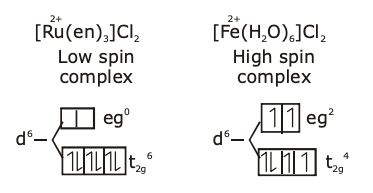Question 14: Consider the following molecules and statements related to them: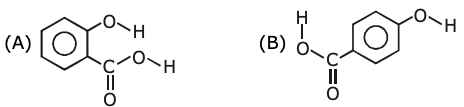(a) (B) is more likely to be crystalline than (A)

(b) (B) has a higher boiling point than (A)

(c) (B) dissolves more readily than (A) in water

Identify the correct option from below:

1) (a) and (c) is true
2) only (a) is true (3) (b) and
3) are true
4) (a) and (b) are true

Question 15: The strengths of 5.6 volume hydrogen peroxide (of density 1 g/mL) in terms of mass percentage and molarity (M), respectively, are: (Take the molar mass of hydrogen peroxide as 34 g/mol)
1) 0.85 and 0.5
2) 0.85 and 0.25
3) 1.7 and 0.25
4) 1.7 and 0.5

Volume strength = 5.6V

Molarity = [5.6 / 11.2] = 0.5 mol / l

Mass % = [(0.5 × 34) / (10)] × [(1) / (1g / ml)]

= 1.7%

The answers are 1.7 & 0.5 that is option (4).

Question 16: The compound A in the following reactions is: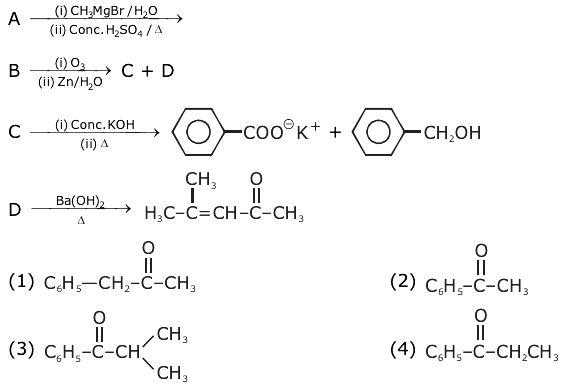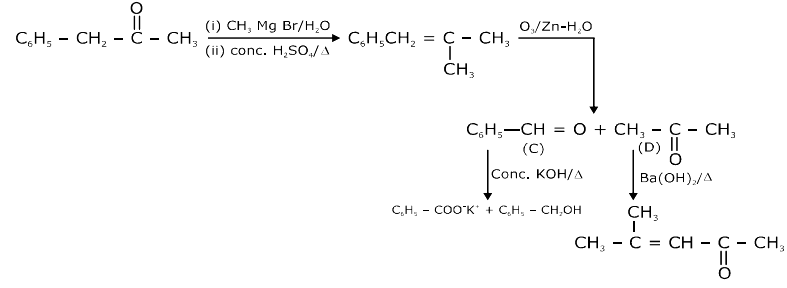Question 17: A mixture of one mole each of H2, He and O2 each are enclosed in a cylinder of volume V at temperature T. If the partial pressure of H2 is 2 atm, the total pressure of the gases in the cylinder is:
1) 6 atm
2) 14 atm
3) 38 atm
4) 22 atm

pH2 = 2 atm = xH2 × ptotal

2 atm = [(1) / (1 + 1 + 1] × ptotal

ptotal = 6 atm

Question 18: Three isomers A, B and C (mol. formula C8H11N) give the following results: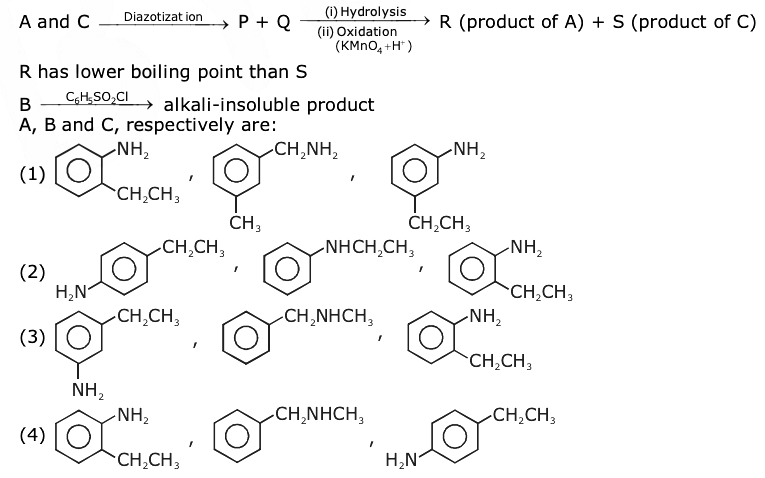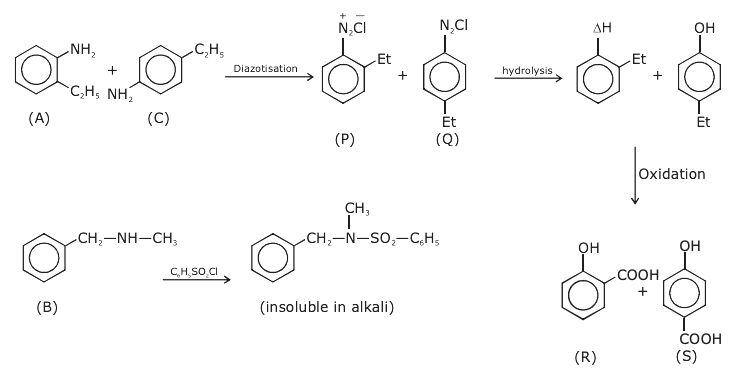Question 19: For the reaction 2A + 3B + (3 / 2) C → 3P, which statement is correct?
1) dnA / dt = dnB / dt = dnC / dt
2) dnA / dt = (3 / 2) dnB / dt = (3 / 4) dnC / dt
3) dnA / dt = (2 / 3) dnB / dt = (4 / 3) dnC / dt
4) dnA / dt = (2 / 3) dnB / dt = (3 / 4) dnC / dt

2A + 3B + (3 / 2) C → 3P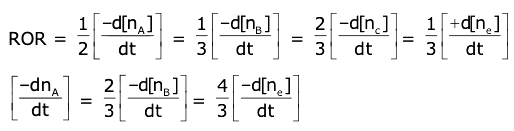Question 20: Consider the following reaction: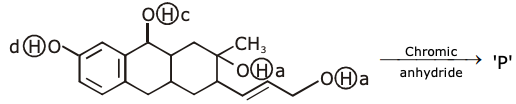The product ‘P’ gives positive ceric ammonium nitrate test. This is because of the presence of which of these –OH group(s)?

1) (b) only
2) (b) and (d)
3) (c) and (d)
4) (d) only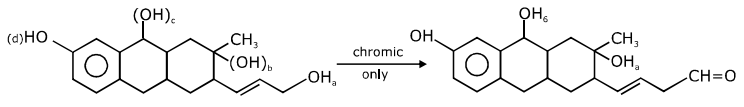Question 21: The volume (in mL) of 0.1 N NaOH required to neutralise 10 mL of 0.1 N phosphinic acid is ___________.

NaOH + H3 PO2 NaH2PO2 + H2O

Phosphinic

Volume × 0.1 = 0.1 × 10

Volume = 10 ml

Question 22: An acidic solution of dichromate is electrolyzed for 8 minutes using 2A current. As per the following equation

Cr2O72- + 14H+ + 6e → 2Cr3+ + + 7H2O

The amount of Cr3+ obtained was 0.104 g. The efficiency of the process (in %) is (Take: F = 96000 C, At. mass of chromium = 52) _______.

[moles of Cr3+] × 3 = [8 × 60 × 2] / 96000

Moles of Cr3+ = [8 × 4] / 9600 = (1 / 300) mol

Mass of Cr3+ = (52 / 300) g

% efficiency = {[Actual obtained amount] / [Theoretical obtained amount]} * 100

= [(0.104 / (52 / 100)) * 100]

= 30 * (104 / 52)

= 60%

Question 23: If 250 cm3 of an aqueous solution containing 0.73 g of a protein A is isotonic with one litre of another aqueous solution containing 1.65 g of a protein B, at 298 K, the ratio of the molecular masses of A and B is ______ × 10–2 (to the nearest integer).

[0.73 / MA] × [1000 / 250] = 1.65 / MB

[MA / MB] = [73 × 4] / 165 = 1.769

= 176.9 × 10–2

= 177 × 10–2

Question 24: 6.023 × 1022 molecules are present in 10 g of a substance ‘x’. The molarity of a solution containing 5 g of substance ‘x’ in 2 L solution is _____ × 10–3.

Mol. wt of ‘x’ = {10 / [6.023 × 1022]} × 6.023 × 1023

M = [5 / 100] / 2 = [(5 / 200) * 1000] × 10-3

M = 25 × 10–3 mol/lit

Question 25: The number of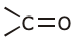groups present in a tripeptide Asp–Glu–Lys is ____.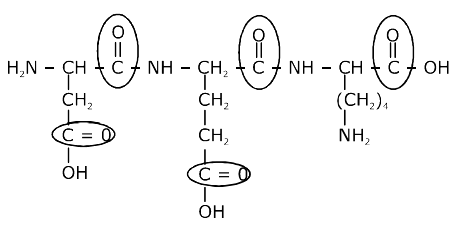## Video Lessons – September 3 Shift 2 Chemistry